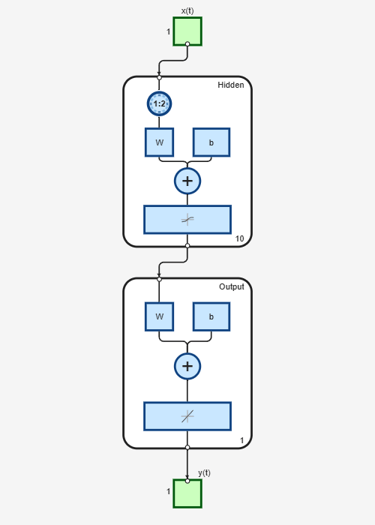# timedelaynet

Time delay neural network

## Syntax

``timedelaynet(inputDelays,hiddenSizes,trainFcn)``

## Description

example

````timedelaynet(inputDelays,hiddenSizes,trainFcn)` takes these arguments: Row vector of increasing 0 or positive input delays, `inputDelays`Row vector of one or more hidden layer sizes, `hiddenSizes`Training function, `trainFcn` and returns a time delay neural network. Time delay networks are similar to feedforward networks, except that the input weight has a tap delay line associated with it. This allows the network to have a finite dynamic response to time series input data. This network is also similar to the distributed delay neural network (`distdelaynet`), which has delays on the layer weights in addition to the input weight.```

## Examples

collapse all

This example shows how to train a time delay network.

Partition the training set. Use `Xnew` to do prediction in closed loop mode later.

```[X,T] = simpleseries_dataset; Xnew = X(81:100); X = X(1:80); T = T(1:80);```

Train a time delay network, and simulate it on the first 80 observations.

```net = timedelaynet(1:2,10); [Xs,Xi,Ai,Ts] = preparets(net,X,T); net = train(net,Xs,Ts,Xi,Ai);````view(net)`Calculate the network performance.

```[Y,Xf,Af] = net(Xs,Xi,Ai); perf = perform(net,Ts,Y);```

Run the prediction for 20 timesteps ahead in closed loop mode.

```[netc,Xic,Aic] = closeloop(net,Xf,Af); view(netc)````y2 = netc(Xnew,Xic,Aic);`

## Input Arguments

collapse all

Zero or positive input delays, specified as an increasing row vector.

Sizes of the hidden layers, specified as a row vector of one or more elements.

Training function name, specified as one of the following.

Training FunctionAlgorithm
`'trainlm'`

Levenberg-Marquardt

`'trainbr'`

Bayesian Regularization

`'trainbfg'`

BFGS Quasi-Newton

`'trainrp'`

Resilient Backpropagation

`'trainscg'`

`'traincgb'`

`'traincgf'`

`'traincgp'`

`'trainoss'`

One Step Secant

`'traingdx'`

`'traingdm'`

`'traingd'`

Example: For example, you can specify the variable learning rate gradient descent algorithm as the training algorithm as follows: `'traingdx'`
Data Types: `char`